# NCERT Solution of Class-11 chemistry Chapter 2- Structure of atom

NCERT Solutions for class-11 Chemistry Chapter 2 Structure of Atom is prepared by our senior and renown teachers of Physics Wallah primary focus while solving these questions of class-11 in NCERT textbook, also do read theory of this Chapter 2 Structure of Atom while going before solving the NCERT questions. Our Physics Wallah team Prepared Other Subjects NCERT Solutions for class 11.

### Chapter 2 Structure of Atom

1. (i) Calculate the number of electrons which will together weigh  one gram.

(ii) Calculate the mass and charge of one mole of electrons.

Solution :

(i) Mass of one electron = 9.10939 × 10–31 kg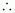Number of electrons that weigh 9.10939 × 10–31 kg = 1

Number of electrons that will weigh 1 g = (1 × 10–3 kg)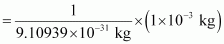= 0.1098 × 10–3 + 31

= 0.1098 × 1028

= 1.098 × 1027

(ii) Mass of one electron = 9.10939 × 10–31 kg

Mass of one mole of electron = (6.022 × 1023) × (9.10939 ×10–31 kg)

= 5.48 × 10–7 kg

Charge on one electron = 1.6022 × 10–19 coulomb

Charge on one mole of electron = (1.6022 × 10–19 C) (6.022 × 1023)

= 9.65 × 104 C

2. (i) Calculate the total number of electrons present in one mole of methane.

(ii) Find (a) the total number and (b) the total mass of neutrons in 7 mg of 14C. (Assume that mass of a neutron = 1.675 × 10–27 kg).

(iii) Find (a) the total number and (b) the total mass of protons in 34 mg of NH3 at STP. Will the Sol. change if the temperature and pressure are changed?

Solution :

(i) Number of electrons present in 1 molecule of methane (CH4)

{1(6) + 4(1)} = 10

Number of electrons present in 1 mole i.e., 6.023 × 1023 molecules of methane

= 6.022 × 1023 × 10 = 6.022 × 1024

(ii) (a) Number of atoms of 14C in 1 mole= 6.023 × 1023

Since 1 atom of 14C contains (14 – 6) i.e., 8 neutrons, the number of neutrons in 14 g of 14C is (6.023 × 1023) ×8. Or, 14 g of 14C contains (6.022 × 1023 × 8) neutrons.

Number of neutrons in 7 mg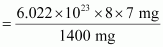= 2.4092 × 1021

(b) Mass of one neutron = 1.67493 × 10–27 kg

Mass of total neutrons in 7 g of 14C

= (2.4092 × 1021) (1.67493 × 10–27 kg)

= 4.0352 × 10–6 kg

(iii) (a) 1 mole of NH3 = {1(14) + 3(1)} g of NH3

= 17 g of NH3

= 6.022× 1023 molecules of NH3

Total number of protons present in 1 molecule of NH3

= {1(7) + 3(1)}

= 10

Number of protons in 6.023 × 1023 molecules of NH3

= (6.023 × 1023) (10)

= 6.023 × 1024

⇒ 17 g of NH3 contains (6.023 × 1024) protons.

Number of protons in 34 mg of NH3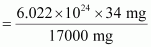= 1.2046 × 1022

(b) Mass of one proton = 1.67493 × 10–27 kg

Total mass of protons in 34 mg of NH3

= (1.67493 × 10–27 kg) (1.2046 × 1022)

= 2.0176 × 10–5 kg

The number of protons, electrons, and neutrons in an atom is independent of temperature and pressure conditions. Hence, the obtained values will remain unchanged if the temperature and pressure is changed.

3. How many neutrons and protons are there in the following nuclei?

136C, 168O , 2412Mg ,5626Fe, 8838Sr

Solution :

136C:

Atomic mass = 13

Atomic number = Number of protons = 6

Number of neutrons = (Atomic mass) – (Atomic number)

= 13 – 6 = 7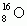:

Atomic mass = 16

Atomic number = 8

Number of protons = 8

Number of neutrons = (Atomic mass) – (Atomic number)

= 16 – 8 = 8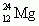:

Atomic mass = 24

Atomic number = Number of protons = 12

Number of neutrons = (Atomic mass) – (Atomic number)

= 24 – 12 = 12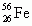:

Atomic mass = 56

Atomic number = Number of protons = 26

Number of neutrons = (Atomic mass) – (Atomic number)

= 56 – 26 = 30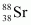:

Atomic mass = 88

Atomic number = Number of protons = 38

Number of neutrons = (Atomic mass) – (Atomic number)

= 88 – 38 = 504. Write the complete symbol for the atom with the given atomic number (Z) and Atomic mass (A)

(i) Z = 17, A = 35

(ii) Z = 92, A = 233

(iii) Z = 4, A = 9

Solution :

(i)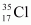(ii)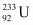(iii)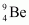5. Yellow light emitted from a sodium lamp has a wavelength (λ) of 580 nm. Calculate the frequency (ν) and wave number (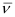) of the yellow light.

Solution :

From the expression,

We get,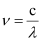…….. (i)

Where,

ν = frequency of yellow light

c = velocity of light in vacuum = 3 × 10m/s

λ = wavelength of yellow light = 580 nm = 580 × 10–9 m

Substituting the values in expression (i):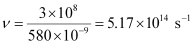Thus, frequency of yellow light emitted from the sodium lamp

= 5.17 × 1014 s–1

Wave number of yellow light,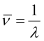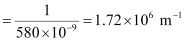6. Find energy of each of the photons which

(i) correspond to light of frequency 3× 1015 Hz.

(ii) have wavelength of 0.50 Å.

Solution :

(i) Energy (E) of a photon is given by the expression,

E =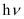Where,

h = Planck’s constant = 6.626 × 10–34 Js

ν = frequency of light = 3 × 1015 Hz

Substituting the values in the given expression of E:

E = (6.626 × 10–34) (3 × 1015)

E = 1.988 × 10–18 J

(ii) Energy (E) of a photon having wavelength (λ) is given by the expression,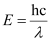h = Planck’s constant = 6.626 × 10–34 Js

c = velocity of light in vacuum = 3 × 108 m/s

Substituting the values in the given expression of E: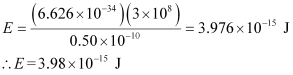7. Calculate the wavelength, frequency and wave number of a light wave whose period is 2.0 × 10–10 s.

Solution :

Frequency (ν) of light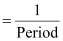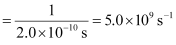Wavelength (λ) of light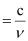Where,

c = velocity of light in vacuum = 3×10m/s

Substituting the value in the given expression of λ: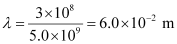Wave number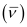of light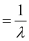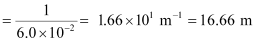8. What is the number of photons of light with a wavelength of 4000 pm that provide 1 J of energy?

Solution :

Energy (E) of a photon = hν

Energy (En) of ‘n’ photons = nhν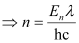Where,

λ = wavelength of light = 4000 pm = 4000 ×10–12 m

c = velocity of light in vacuum = 3 × 108 m/s

h = Planck’s constant = 6.626 × 10–34 Js

Substituting the values in the given expression of n: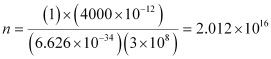Hence, the number of photons with a wavelength of 4000 pm and energy of 1 J are 2.012 × 1016.

9. A photon of wavelength 4 × 10–7 m strikes on metal surface, the work function of the metal being 2.13 eV. Calculate (i) the energy of the photon (eV), (ii) the kinetic energy of the emission, and (iii) the velocity of the photoelectron (1 eV= 1.6020 × 10–19 J).

Solution :

(i) Energy (E) of a photon = hν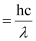Where,

h = Planck’s constant = 6.626 × 10–34 Js

c = velocity of light in vacuum = 3 × 10m/s

λ = wavelength of photon = 4 × 10–7 m

Substituting the values in the given expression of E: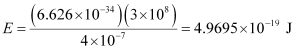Hence, the energy of the photon is 4.97 × 10–19 J.

(ii) The kinetic energy of emission Ek is given by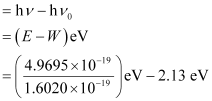= (3.1020 – 2.13) eV

= 0.9720 eV

Hence, the kinetic energy of emission is 0.97 eV.

(iii) The velocity of a photoelectron (ν) can be calculated by the expression,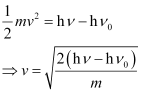Where,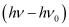is the kinetic energy of emission in Joules and ‘m’ is the mass of the photoelectron. Substituting the values in the given expression of v: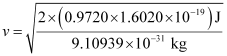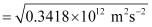v = 5.84 × 105 ms–1

Hence, the velocity of the photoelectron is 5.84 × 105 ms–1.

10. Electromagnetic radiation of wavelength 242 nm is just sufficient to ionise the sodium atom. Calculate the ionisation energy of sodium in kJ mol–1.

Solution :

Energy of sodium (E)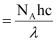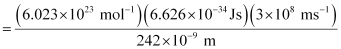= 4.947 × 105 J mol–1

= 494.7 × 103 J mol–1

= 494 kJ mol–1

11. A 25 watt bulb emits monochromatic yellow light of wavelength of 0.57μm. Calculate the rate of emission of quanta per second.

Solution :

Power of bulb, P = 25 Watt = 25 Js–1

Energy of one photon, E = hνSubstituting the values in the given expression of E: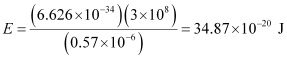E = 34.87 × 10–20 J

Rate of emission of quanta per second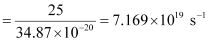12. Electrons are emitted with zero velocity from a metal surface when it is exposed to radiation of wavelength 6800 Å. Calculate threshold frequency (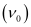) and work function (W0) of the metal.

Solution :

Threshold wavelength of radian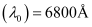= 6800 × 10–10 m

Threshold frequencyof the metal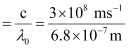= 4.41 × 1014 s–1

Thus, the threshold frequencyof the metal is 4.41 × 1014 s1.

Hence, work function (W0) of the metal = hν0

= (6.626 × 10–34 Js) (4.41 × 1014 s–1)

= 2.922 × 10–19 J

13. What is the wavelength of light emitted when the electron in a hydrogen atom undergoes transition from an energy level with n = 4 to an energy level with n = 2?

Solution :

The ni = 4 to nf = 2 transition will give rise to a spectral line of the Balmer series. The energy involved in the transition is given by the relation,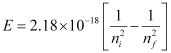Substituting the values in the given expression of E: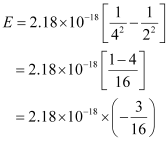E = – (4.0875 × 10–19 J)

Wavelength of light emitted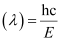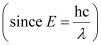Substituting the values in the given expression of λ: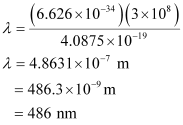14. How much energy is required to ionise a H atom if the electron occupies n = 5 orbit? Compare your Sol. with the ionization enthalpy of H atom (energy required to remove the electron from n =1 orbit).

Solution :

The expression of energy is given by,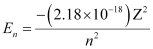Where,

Z = atomic number of the atom

n = principal quantum number

For ionization from n1 = 5 to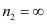,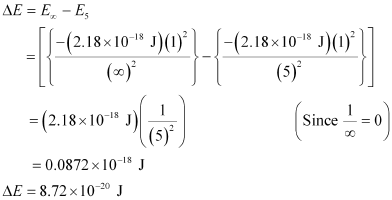Hence, the energy required for ionization from n = 5 to n =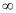is 8.72 × 10–20 J.

Energy required for n1 = 1 to n =,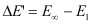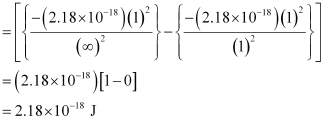Hence, less energy is required to ionize an electron in the 5th orbital of hydrogen atom as compared to that in the ground state.

15. What is the maximum number of emission lines when the excited electron of a H atom in n = 6 drops to the ground state?

Solution :

The number of spectral lines produced when an electron in the nthlevel drops down to the ground state is given by n(n-1)/2.

Given, n=6

∴ Number of spectral lines = 6×5/2 = 15

also given by, ∑(n2 - n1) = ∑(6-1) = ∑5 = 5+4+3+2+1 = 15

16. (i) The energy associated with the first orbit in the hydrogen atom is –2.18×10-18 J atom-1. What is the energy associated with the fifth orbit? (ii) Calculate the radius of Bohr’s fifth orbit for hydrogen atom.

Solution :

(i) En = -21.8×10-19/n2 J

∴ E= -21.8×10-18/52 J = 8.72×10-20 J

(ii) For H atom, rn = 0.529×nÅ

∴  r= 0.529×5= 13.225 Å = 1.3225 nm

17. Calculate the wavenumber for the longest wavelength transition in the Balmer series of atomic hydrogen.

Solutions :

For the Balmer series, n1 = 2. Hence, ṽ = R(1/2- 1/n22)
ṽ = 1/λ  (inversely proportional)
For λ to be maximum, ṽ should be minimum. This can be happened when n2 is minimum i.e. n2 = 3. Hence, ṽ = (1.097×10m-1) (1/2- 1/32) = 1.097×107×5/36 m-1 = 1.523×10m-1

18. What is the energy in joules, required to shift the electron of the hydrogen atom from the first Bohr orbit to the fifth Bohr orbit and what is the wavelength of the light emitted when the electron returns to the ground state? The ground state electron energy is –2.18×10-11  ergs.

Solution :
1erg = 10-7 J
As ground state electronic energy is  –2.18 ×10-11 ergs, this means that En = -21.8 ×10-11/n2 ergs.

ΔE =  E5  - E=  2.18×10-11  (1/1- 1/52) = 2.18 ×10-11 (24/25) = 2.09 × 10-11 ergs =  2.09×10-18 J

When electron returns to ground state (n=1), energy emitted = 2.09×10-11 ergs.

As, E = hν = hc/λ

⇒ λ = hc/E = (6.626  × 10-27 erg sec) (3.0 × 1010 cm s-1 ) / 2.09 × 10-11 ergs

= 9.51 × 10-6 cm = 951 × 10-8 cm = 951 Å

19. The electron energy in hydrogen atom is given by E n = (–2.18×10-18) /n2 J. Calculate the energy required to remove an electron completely from the n = 2 orbit. What is the longest wavelength of light in cm that can be used to cause this transition?

Solution :
ΔE =  E-  E2 = 0 – (–2.18 ×10-18 J atom-1/22) = 5.45 × 10-19 J atom-1
ΔE = hν = hc/λ
⇒ λ = hc/ΔE  = (6.626 × 10-34 Js) × (3.0 × 10ms-1)/ 5.45 × 10-19 J = 3.674 × 10-7 m = 3.674 × 10-5 cm

20. Calculate the wavelength of an electron moving with a velocity of 2.05×107 ms-1.

Solution :

By de Broglie equation,

λ  = h/mv = 6.626 × 10-34 Js/ (9.11×10-31 kg)  (2.05×107 ms-1)  = 3.55 ×10-11 m

21. The mass of an electron is 9.11×10-31 kg. If its K.E. is 3.0×10-25 J, calculate its wavelength.

Solution :
K.E. = 1/2 mv2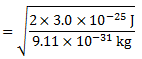∴ v = √2 K.E./m

= 812 ms-1 By de Broglie equation, λ = h/mv = 6.626 × 10-34 Js/ (9.11 × 10-31 kg) (812 ms-1) = 8.967 ×10-7 m

22. Which of the following are isoelectronic species i.e., those having the same number of electrons?
Na+ , K+ , Mg2+ , Ca2+ , S2- , Ar.

Solution :
Notes: Isoelectronic are the species having same number of electrons.
A positive charge means the shortage of an electron.
A negative charge means gain of electron.
Number of electrons in Na+ = 11-1 = 10
Number of electrons in K+ = 19-1 = 18
Number of electrons in Mg2+ = 12-2 = 10
Number of electrons in Ca2+ = 20-2 = 18
Number of electrons in S2- = 16+2 = 18
Number of electrons in Ar = 18
Hence, the following are isoelectronic species:
1) Na+ andMg2+ ( 10 electrons each )
2) K+, Ca2+, S2- and  Ar ( 18 electrons each )

23. (i) Write the electronic configurations of the following ions: (a) H- (b) Na+ (c) O2- (d) F-
(ii) What are the atomic numbers of elements whose outermost electrons are represented by (a) 3s1 (b) 2pand (c) 3p5 ?
(iii) Which atoms are indicated by the following configurations ? (a) [He]2s(b) [Ne] 3s3p3 (c) [Ar] 4s2 3d1 .

Solution :
(i) (a) 1H = 1s1 . A negative charge means gain of electron.
∴ electronic configuration of H= 1s2
(b) 11Na = 1s22s2 2p63s1 . A positive charge means the shortage of an electron.
∴ electronic configuration of Na= 1s22s22p6
(c) 8O = 1s22s22p4
∴ electronic configuration of O2- = 1s2 2s2 2p6 (d) 9F = 1s2 2s2 2p5
∴ electronic configuration of F- = 1s2 2s2 2p6 (ii) (a) 3s1
Completing the electron  configuration  of the element as 1s2 2s2 2p6 3s1
∴ Number of electrons present in the atom of the element = 2+2+6+1 = 11
∴ Atomic number of the element = 11
(b) 2p3
Completing the electron configuration of the element as 1s22s22p3
∴ Number of electrons present in the atom of the element = 2+2+3 = 7
∴ Atomic number of the element = 7
(c) 3p5
Completing the electron configuration of the element as 1s2 2s2 2p6 3s23p5
∴ Number of electrons present in the atom of the element = 2+2+6+2+5 = 17
∴ Atomic number of the element = 9
(iii)  (a) [He]2s1
electronic configuration = 1s22s1
∴ Atomic number of the element = 2+1 = 3
Hence, the element with the electronic configuration  [He] 2s1 is lithium (Li).
(b) [Ne] 3s23p3
electronic configuration = 1s2 2s2 2p6 3s2 3p3
∴ Atomic number of the element = 2+2+6+2+3 = 15
Hence, the element with the electronic configuration  [Ne] 3s23p3 is phosphorus (P).
(c) [Ar] 4s23d1
electronic configuration = 1s2 2s2 2p6 3s2 3p6 4s2 3d1
∴ Atomic number of the element = 2+2+ 6+2+ 6+2+1 = 21
Hence, the element with the electronic configuration [Ar] 4s23d1 is scandium (Sc).

24. What is the lowest value of n that allows g orbitals to exist?

Solution :
For g-orbitals, l = 4.
For any value 'n' of principal quantum number, the Azimuthal quantum number (l) can have a value from zero to (n - 1).
∴ For l = 4, minimum value of n = 5

25. An electron is in one of the 3d orbitals. Give the possible values of n, l and mfor this electron.

Solution :
For the 3d orbital:
Principal quantum number (n) = 3
Azimuthal quantum number (l) = 2
Magnetic quantum number (ml) = -2,-1,0,1,2

26. An atom of an element contains 29 electrons and 35 neutrons. Deduce (i) the number of protons and (ii) the electronic configuration of the element.

Solution :
(i) For  neutral atom,  number of protons = number of electrons.
∴ Number of protons in the atom of the given element = 29 = Atomic number
(ii) The electronic configuration of the atom with Z=29 is  1s2  2s2  2p6  3s 3p4s1 3d10

27. Give the number of electrons in the species H2, H2 and O2+

Solution :
H2= 2-1 = 1 electron
H2 = 1H + 1H = 2 electrons
O2= 16-1 = 15 electrons

28. (i) An atomic orbital has n = 3. What are the possible values of l and ml ?
(ii) List the quantum numbers (ml and l ) of electrons for 3d orbital.
(iii) Which of the following orbitals are possible?
1p, 2s, 2p and 3f

Solution :
(i) For a given value of n, l can have values from 0 to (n-1).
∴ For n = 3 , l = 0, 1, 2
For a given value of l, ml can have (2l+1) values.
When l = 0, m = 0
l = 1, m = - 1, 0, 1
l = 2, m = - 2, - 1, 0, 1, 2
l = 3, m = -3, -2, -1, 0, 1, 2, 3
(ii) For 3d orbital, n = 3, l = 2.
∴ For l = 2
m2 = -2, -1, 0, 1, 2
(iii) 1p is not possible because when n = 1, l = 0. (for p, l = 1)
2s is possible because when n=2, l = 0,1 (for s, l=0)
2p is possible because when n=2, l = 0,1 (for p, l=1)
3f is not possible because when n=3, l = 0, 1 , 2 (for f, l=3)

29. Using s, p, d notations, describe the orbital with the following quantum numbers.
(a) n=1, l=0; (b) n=3; l=1 (c) n=4; l=2; (d) n=4; l=3.

Solution :
(a) 1s
(b) 3p
(c) 4d
(d) 4f

30. Explain, giving reasons, which of the following sets of quantum numbers are not possible.
(a) n = 0,           l = 0,         ml = 0,            ms = +½
(b) n = 1,          l = 0,          ml = 0,            ms = –½
(c) n = 1,          l = 1,          ml = 0,            ms = +½
(d) n = 2,          l = 1,          ml = 0,            ms = –½
(e) n = 3,          l = 3,          ml = –3,          ms = +½
(f) n = 3,          l = 1,           ml = 0,            ms = +½

Solution :
(a) Not possible because n≠0
(b) Possible
(c) not possible because when n=1, l≠1
(d) Possible
(e) Not possible because when n=3,  l≠3
(f) Possible

31. How many electrons in an atom may have the following quantum numbers?
(a) n = 4, ms = –½        (b) n = 3, l = 0

Solution :
(i) The total number of electrons in n is given by 2n2
n=4, Number of electrons = 2×4= 32
Half of 32 electrons will have spin quantum number ms = –½ i.e. 16 electrons
(ii) n=3 and l=0 means it is 3s orbital which can have only 2 electrons.

32. Show that the circumference of the Bohr orbit for the hydrogen atom is an integral multiple of the de Broglie wavelength associated with the electron revolving around the orbit.

Solution :
According to Bohr postulate of angular momentum,
mvr = nh/2π ⇒ 2πr = nh/mv   ...(i)
According to de Broglie equation, λ=h/mv   ...(ii)
Substituting the value of eqn (ii) in eqn (i) we get,
2πr = nλ
Thus, circumference of the Bohr orbit for the hydrogen atom is an integral multiple of de Broglie's wavelength associated with the electron revolving around the orbit.

33. What transition in the hydrogen spectrum would have the same wavelength as the Balmer transition n = 4 to n = 2 of He+ spectrum?

Solution :
For H-like particles, ṽ = (2π2 mZ2 e4/ ch3)×(1/n1- 1/n22) = RZ2(1/n1- 1/n22)
∴ For He+ spectrum, Balmer transition, n=4 to n=2
ṽ = 1/λ = RZ2(1/2- 1/42)  = R×4×3/16 = 3R/4
For hydrogen spectrum ,
ṽ = 1/λ = R(1/n1- 1/n22) = 3R/4  ⇒ (1/n1- 1/n22) = 3/4
which can be true only for n1=1 and n2=2 i.e. transition from n=2 to n=1.

34. Calculate the energy required for the process
He+(g) →  He2+ (g) + e
The ionization energy for the H atom in the ground state is 2.18×10-18 J atom-1.

Solution :
For H-like particles, E= - (2π2 mZ2e4 /n2h2)
For H-atom, I.E. = E-E= 0 - (-2π2m × 22× e4/12×h2
= (4×2π2 me4/h2) = 4×2.18 × 10-18 J = 8.72 × 10-18 J

35. If the diameter of a carbon atom is 0.15 nm, calculate the number of carbon atoms which can be placed side by side in a straight line across length of scale of length 20 cm long.

Solution :
Diameter of a carbon atom = 0.15 nm = 0.15 ×10-9 m = 1.5 × 10-10 m
Length along which atoms are to be placed = 20 cm = 2 × 10-1 m
∴ No. of C-atoms which can be placed along the line = 2 × 10-1 m/1.5 × 10-10 m = 1.33 × 109

36. 2×108 atoms of carbon are arranged side by side. Calculate the radius of carbon atom if the length of this arrangement is 2.4 cm.

Solution :
Total length = 2.4 cm
Total number of atoms along the length = 2 ×108
∴ Diameter of each atom = 2.4 cm/2 × 108 = 1.2×10-8 cm
∴ Radius of the atom = Diameter/2 = 1.2 × 10-8 cm/2 = 0.6×10-8 cm

37. The diameter of zinc atom is 2.6 Å.Calculate (a) radius of zinc atom in pm and (b)number of atoms present in a length of 1.6 cm if the zinc atoms are arranged side by side lengthwise.

Solution :
(a) Radius of zinc atom = 2.6Å /2 = 1.3Å = 1.3 × 10-10 m = 130 ×10-12 pm
(b) Given length = 1.6 cm = 1.6 ×10-2 m
Diameter of one atom = 2.6 Å = 2.6 × 10-10 m
∴ No. of atoms present along the length = 1.6 × 10-2/ 2.6 × 10-10 = 6.154 × 107

38. A certain particle carries 2.5 × 10-16 C of static electric charge. Calculate the number of electrons present in it.

Solution :
Charge on one electron = 1.602 × 10-19 C
∴ Number of electrons carrying 2.5 × 10-16 C charge = 2.5 × 10-16/ 1.602 × 10-19 =1560

39. In Milikan’s experiment, static electric charge on the oil drops has been obtained by shining  X-rays. If the static electric charge on the oil drop is –1.282×10-18 C, calculate the number of electrons present on it.

Solution :
As in the above question,
Number of electrons present in oil drop = –1.282 × 10-18/ 1.602×10-19 = 8

40. In Rutherford’s experiment, generally the thin foil of heavy atoms, like gold, platinum etc. have been used to be bombarded by the α-particles. If the thin foil of light atoms like aluminium etc. is used, what difference would be observed from the above results ?

Solution :
Heavy atoms have nucleus carrying  large amount of positive charge. Therefor, some α-particles will easily deflected back. Also a number of α-particles are deflected through small angles because of large positive charge.
If light atoms are used, their nuclei will have small positive charge, hence the number of α-particles getting deflected even through small angles will be negligible.

41. Symbols 79Br35 and 79Br can be written, whereas symbols  35Br79 and 35Br are not acceptable .  Answer  briefly.

Solution :
35Br79 is not acceptable because atomic number should be written as subscript, while mass number should be written as superscript. 35Br is not acceptable because atomic number of an element is fixed. However, mass number is not fixed as it depends upon the isotopes taken. Hence, it is essential to indicate mass number.

42. An element with mass number 81 contains 31.7% more neutrons as compared to protons. Assign the atomic symbol.

Solution :
Mass number = protons+neutrons = p + n = 81 (given)
Let p be x, then neutrons = x + (31.7 /100)x = 1.317 x
∴ x + 1.317 x = 81
⇒ 2.317 x = 81
⇒ x = 81/2.317 = 35
Thus, protons = 35 = atomic number.
The symbol of the element is 81Br35  or 8135Br

43. An ion with mass number 37 possesses one unit of negative charge. If the ion contains 11.1% more neutrons than the electrons, find the symbol of the ion.

Solution :
Let the number of electrons in the ion = x
Then, number of neutrons = x+(11.1 x/ 100) = 1.111 x
Number of electrons in the neutral atom = x-1 (ion possesses one unit of negative charge)
∴ Number of protons = x-1
Mass number = No. of protons + No. of neutrons
∴ 1.111 x + x - 1 = 37
⇒ 2.111x = 38
⇒ x = 18
∴ No. of protons = Atomic no. = x-1 = 18-1 = 17
The symbol of the ion is 3717Cl-1

44. An ion with mass number 56 contains 3 units of positive charge and 30.4% more neutrons than electrons. Assign the symbol to this ion.

Solution :
Let the number of electrons in the ion = x
Then, number of neutrons = x+(30.4 x/ 100) = 1.304 x
Number of electrons in the neutral atom = x+3 (ion possesses 3 units of positive charge)
∴ Number of protons = x+3
Mass number = No. of protons + No. of neutrons
∴ 1.304 x + x +3 = 56
⇒ 2.304x = 53
⇒ x = 23
∴ No. of protons = Atomic no. = x+3 = 23+3 = 26
The symbol of the ion is 5626Fe+3

45. Arrange the following type of radiations in increasing order of frequency: (a) radiation from microwave oven (b) amber light from traffic signal (c) radiation from FM radio (d) cosmic rays from outer space and (e) X-rays.

Solution :
The increasing order of frequency is as follows:
Radiation from FM radio < amber light < radiation from microwave oven < X- rays < cosmic rays

46. Nitrogen laser produces a radiation at a wavelength of 337.1 nm. If the number of photons emitted is 5.6×1024, calculate the power of this laser.

Solution :
E = Nhν = Nhc/λ = (5.6 ×1024) × (6.626 × 10-34 Js × 3.0×10ms-1) /337.1 × 10-9 m = 3.3 × 10J

47. Neon gas is generally used in the sign boards. If it emits strongly at 616 nm, calculate (a) the frequency of emission, (b) distance traveled by this radiation in 30 s (c) energy of quantum and (d) number of quanta present if it produces 2 J of energy.

Solution :
λ  = 616 nm = 616 × 10-9 m
(a) Frequency, ν = c/λ = 3.0 ×10ms-1 / 616 × 10-9 m = 4.87× 1014 s-1 (b) Velocity of the radiation = 3.0×10ms-1
∴ Distance travelled in 30 s = 30×3 × 10m = 9.0×10m
(c) E = hν = hc/λ = (6.626 × 10-34 Js × 3.0 × 10ms-1) / 616×10-9 m = 32.27×10-20 J
(d) No. of quanta in 2J of energy = 2J /32.27 × 10-20 J = 6.2 × 1018

48. In astronomical observations, signals observed from the distant stars are generally weak. If the photon detector receives a total of 3.15×10-18 J from the radiations of 600 nm, calculate the number of photons received by the detector.

Solution :
Energy of one photon = hν = hc/λ = (6.626 × 10-34 Js × 3.0 × 10ms-1) / 600 × 10-9 m = 3.313 × 10-19 J
Total energy received = 3.15 × 10-18 J
∴ No. of photons received = 3.15 × 10-18 J/3.313 × 10-19 J = 9.51 (approx 10)

49. Lifetimes of the molecules in the excited states are often measured by using pulsed radiation source of duration nearly in the nano second range. If the radiation source has the duration of 2 ns and the number of photons emitted during the pulse source is 2.5×1015 , calculate the energy of the source.

Solution :
Frequency = 1/2 ×10-19 s =  0.5 ×10s-1 Energy = Nhν = (2.5 × 1015) × (6.626 × 10-34 Js)× (0.5 ×10s-1)  = 8.28 ×10-10 J

50. The longest wavelength doublet absorption transition is observed at 589 and 589.6 nm. Calcualte the frequency of each transition and energy difference between two excited states.

Solution :
λ1 = 589 nm = 589 ×10-9 m
∴ ν1 = c/λ1 = 3.0 × 10ms-1/ 589 × 10-9 m = 5.093 × 1014 s-1
λ2 = 589.6 nm = 589.6 × 10-9 m
∴ ν2 = c/λ2 = 3.0×10ms-1/ 589.6 × 10-9 m = 5.088 × 1014 s-1
ΔE = E2 - E= h( ν- ν1) = (6.626 × 10-34 Js) × (5.093 -5.088) × 1014  s-1  = 3.31 ×10-22 J

51. The work function for caesium atom is 1.9 eV. Calculate (a) the threshold wavelength and (b) the threshold frequency of the radiation. If the caesium element is irradiated with a wavelength 500nm, calculate the kinetic energy and the velocity of the ejected photoelectron.

Solution :
(a) Work function (W0) = hν0
∴ ν= W0/h = 1.9 ×1.602 × 10-19 J/ 6.626 × 10-34 Js = 4.59×1014 s-1            (1eV = 1.602×10-19 J)
(b) λ0 = c/ν= 3.0 ×10ms-1/4.59 ×1014 s-1 = 6.54×10-7 m = 654 ×10-9 m = 654 nm
(c) K.E. of ejected electron = h(ν - ν0) = hc (1/λ - 1/λ)
= (6.626 ×10-34 Js×3.0× 10ms-1)×(1/500 ×10-9 m - 1/654 ×10-9 m)
= (6.626 ×3.0 × 10-26/10-9) ×( 154/500 ×654) J = 9.36 ×10-20 J
K.E. = 1/2 mv= 9.36×10-20 J
∴ 1/2×(9.11 ×10-31 kg) v= 9.36 ×10-20 kgm2s-2
⇒ v= 2.055× 1011 m2s-2 = 20.55 ×1010 m2s-2
⇒ v = 4.53×10ms-1

52. Following results are observed when sodium metal is irradiated with different wavelengths.

Calculate (a) threshold wavelength and, (b) Planck’s constant.

λ (nm)                          500             450           400
v×10-5(cm s-1)               2.55            4.35          5.35

Solution :
Let the threshold wavelength to be λnm = λ0×10-9 m .
Following equation holds true for photoelectric emission in given case:
K.E. = 1/2 mv= h(ν - ν0)
⇒1/2 mv= hν - 0
⇒ hν= hν - 1/2 mv2
⇒ hc/λ0 = hc/λ - 1/2 mv2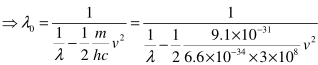(a) Substituting the value of λ and v from the above given data, we get three values of λas,
λ0(1) = 541 nm
λ0(2) = 546 nm
λ0(3) = 542 nm
Threshold frequency = λav = {λ0(1)+ λ0(2)+ λ0(3)}/3 = (541+ 546+ 542)/3 = 543 (approx 540)
(b) Part of this question can't be solved due to incorrect value of v i.e 5.35.
Students can assume this value as 5.20 if they want to solve this question.

53. The ejection of the photoelectron from the silver metal in the photoelectric effect experiment can be stopped by applying the voltage of 0.35 V when the radiation 256.7 nm is used. Calculate the work function for silver metal.

Solution :
Energy of the incident radiation = Work function + Kinetic energy of photoelectron
E = hc/λ = (6.626 × 10-34 Js×3.0 ×10ms-1) / (256.7 × 10-9 m) = 7.74 × 10-19 J = 4.83 eV
The potential applied gives kinetic energy to the electron.
Hence, kinetic energy of the electron = 0.35 eV
∴ Work Function = 4.83 eV - 0.35 eV = 4.48 eV

54. If the photon of the wavelength 150 pm strikes an atom and one of its inner bound electrons is ejected out with a velocity of 1.5×10ms-1 , calculate the energy with which it is bound to the nucleus.

Solution :
Energy of the incident photon= hc/λ = (6.626 ×10-34 Js × 3.0 ×10ms-1) /(150×10-12m) = 13.25 × 10-16 J
Energy of the electron ejected = 1/2 mv= 1/2× (9.11 × 10-31kg) × (1.5×107ms-1)= 1.025 × 10-16 J
Energy with which the electron was bound to the nucleus = 13.25 × 10-16 J - 1.025 × 10-16 J
= 12.225 × 10-16 J = 12.225 × 10-16 / 1.602×10-19 eV = 7.63×10eV

55. Emission transitions in the Paschen series end at orbit n = 3 and start from orbit n and can be represeted as v = 3.29×1015(Hz) [1/32–1/n2] Calculate the value of n if the transition is observed at 1285 nm. Find the region of the spectrum.

Solution :
ν = c/λ = 3.0 ×10ms-1/ 1285 × 10-9 m = 3.29 × 1015 (1/3- 1/n2)
⇒ 1/n= 1/9 - (3.0 ×10ms-1/ 1285×10-9 m) × (1/3.29 × 1015) = 0.111- 0.071 = 0.04 = 1/25
⇒ n= 25
⇒ n = 5
The radiation corresponding to 1285 nm lies in the infrared region.

56. Calculate the wavelength for the emission transition if it starts from the orbit having radius 1.3225 nm and ends at 211.6 pm. Name the series to which this transition belongs and the region of the spectrum.

Solution :
Radius of nth orbit of H-like particles = 0.529n2 /Z Å = 52.9n2/Z pm
r= 1.3225 nm = 1322.5 pm = 52.9n12
r= 211.6 pm = 52.9 n22/Z
∴ r1/r2 = 1322.5 pm / 211.6 pm = n12/n22
⇒ n12/ n2= 6.25
⇒ n1/ n2 = 2.5
If n= 2, n1 = 5. Thus the transition is from 5th orbit to 2nd orbit. It belongs to Balmer series.
ṽ = 1.097 × 10m-1 (1/2- 1/52) = 1.097×107×21/100 m-1
λ = 1/ṽ = 100/ (1.097 ×21 ×107) m = 434 × 10-9 m = 434 nm
It lies in visible range.

57. Dual behaviour of matter proposed by de Broglie led to the discovery of electron microscope often used for the highly magnified images of biological molecules and other type of material. If the velocity of the electron in this microscope is 1.6×10ms-1 , calculate de Broglie wavelength associated with this electron.

Solution :
λ = h/mv = 6.626 ×10-34  kgm2s-1 /(9.11 × 10-31 kg) (1.6 × 10ms-1) = 4.55 × 10-10 m = 455 pm

58. Similar to electron diffraction, neutron diffraction microscope is also used for the determination of the structure of molecules. If the wavelength used here is 800 pm, calculate the characteristic velocity associated with the neutron.

Solution :
Mass of neutron = 1.675 × 10-27 kg
λ = h/mv
⇒ v = h/mλ = 6.626 × 10-34 kgm2s-1/(1.675 ×10-27 kg) (800 ×10-12 m) = 4.94 ×10ms-1

59. If the velocity of the electron in Bohr’s first orbit is 2.19×106 ms-1, calculate the de Broglie wavelength associated with it.

Solution :
λ = h/mv = 6.626×10-34 kgm2s-1/(9.11 × 10-31 kg) (2.19 × 106 ms-1) = 3.32 × 10-10 m =332 pm

60. The velocity associated with a proton moving in a potential difference of 1000 V is 4.37×10ms-1. If the hockey ball of mass 0.1 kg is moving with this velocity, calcualte the wavelength associated with this velocity.

Solution :
λ = h/mv = 6.626 × 10-34 kgm2s-1 /(0.1 kg) (4.37 × 105 ms-1) = 1.516 ×10-28 m

61. If the position of the electron is measured within an accuracy of + 0.002 nm, calculate the uncertainty in the momentum of the electron. Suppose the momentum of the electron is
h/(4π×0.05 nm), is there any problem in defining this value.

Solution :
Δx = 0.002 nm = 2×10-12 m
Δx × Δp = h/4π
∴ Δp = h /4πΔx = 6.626×10-34 kgm2s-1 / (4×3.14×2 ×10-12 m) = 2.638×10-23 kgms-1
Actual momentum = h/(4π×0.05 nm) = h/(4π×5 ×10-11 m)
= 6.626 ×10-34 kgm2s-1/(4×3.14 ×5×10-11 m) = 1.055 ×10-24 kgms-1
It cannot be defined because the actual magnitude of the momentum is smaller than the uncertainty.

62. The quantum numbers of six electrons are given below. Arrange them in order of increasing energies. If any of these combination(s) has/have the same energy lists:
1. n = 4, l = 2, ml  = –2 , m s = –1/2
2. n = 3, l = 2, ml  = 1 , m s = +1/2
3. n = 4, l = 1, ml  = 0 , m s = +1/2
4. n = 3, l = 2, ml  = –2 , m s = –1/2
5. n = 3, l = 1, ml  = –1 , m s = +1/2
6. n = 4, l = 1, ml  = 0 , m s = +1/2

Solution :
The orbitals occupied by the electrons are:
(1) 4d
(2) 3d
(3) 4p
(4) 3d
(5) 3p
(6) 4p
Same orbitals will have same energy and higher the value of (n+l) higher is the energy,
Their energies will be in order: (5)<(2)=(4)<(6)=(3)<(1)

63. The bromine atom possesses 35 electrons. It contains 6 electrons in 2p orbital, 6 electrons in 3p orbital and 5 electron in 4p orbital. Which of these electron experiences the lowest effective nuclear charge ?

Solution :
4p electrons, being farthest from the nucleus experience the lowest effective nuclear charge.

64. Among the following pairs of orbitals which orbital will experience the larger effective nuclear charge? (i) 2s and 3s, (ii) 4d and 4f, (iii) 3d and 3p.

Solution :
(i) 2s is closer to the nucleus than 3s. Hence, 2s will experience larger effective nuclear charge.
(ii) 4d (Reason being the same as above)
(iii) 3p (Reason being the same as above)

65. The unpaired electrons in Al and Si are present in 3p orbital. Which electrons will experience more effective nuclear charge from the nucleus ?

Solution :
Silicon has greater nuclear charge (+14) than aluminium (+13). Hence, the unpaired 3p electron in case of silicon will experience more effective nuclear charge.

66. Indicate the number of unpaired electrons in : (a) P, (b) Si, (c) Cr, (d) Fe and (e) Kr.

Solution :
(a) 15P = 1s2s2p3s3 pxpypz1. 3 unpaired electrons. (in 3p)
(b) 14Si = 1s2s2p3s3 pxpy1. 2 unpaired electrons.(in 3p)
(c) 24Cr = 1s2s2p3s3p3d4s1. 6 unpaired electrons. (5 in 3d and 1 in 4s)
(d)  26Fe = 1s2s2p3s3p3d4s2. 4 unpaired electrons. (in 3d)
(e) 36Kr = It is a Noble gas. All orbitals are filled. No unpaired electrons.

67. (a) How many sub-shells are associated with n = 4 ? (b) How many electrons will be present in the sub-shells having mvalue of –1/2 for n = 4 ?

Solution :
(a) n=4, l = 0, 1, 2, 3. 4 sub-shells are associated with n = 4
(b) No. of orbitals in the shells = n= 4= 16
Each orbitals has one electron with m= -1/2. Hence, there will be 16 electrons with m= -1/2.